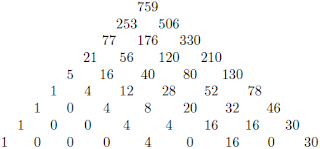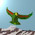## Sunday, January 1, 2012

### Higgsy Codes

Dharwadker's four color theorem uses the Steiner system $S(5,8,24)$, otherwise known as the Witt design. To construct the Steiner system, one starts with the extended binary Golay code. This has $2^{12} = 4096$ length $24$ words, selected from all possible binary words by writing a list of length $24$ binary words so that each word differs from the previous ones in at least $8$ places. It turns out that the code words are partitioned

$4096 = 1 + 759 + 2576 + 759 + 1$

into words with no $1$-bits, words with $8$ $1$-bits, words with $12$ $1$-bits, $16$ $1$-bits and $24$ $1$-bits, respectively. The $729$ words with $8$ $1$-bits form the Steiner system, which is defined as a set of $24$ points, along with the $759$ special subsets of size $8$, called blocks, such that every $5$ points is contained in one of the blocks. The number $759$ is very interesting. It can be partitioned into

$759 = 1 + 280 + 448 + 30$

which counts, respectively, the number of subsets that intersect a given subset in $8$, $4$, $2$ or zero elements. That is, there are $30$ blocks with no intersection with a given block, and $729 = 27^2$ with a non empty intersection. We write $280 = 4 \times 70$, $448 = 16 \times 28$ and $30 = 30 \times 1$, where $70$, $28$ and $1$ are weights for the Reed-Muller code $RM(2,3)$. The $4$, $16$ and $30$ appear in the bottom row of the Pascal triangle below. The number 759 is the summit of this triangle, which counts the number of blocks that (i) contain a point set with $n$ elements and (ii) are disjoint from another set with $m$ elements. The union cardinality $m + n$ is fixed in each row. So there are $253$ occurrences of any given object, and a complementary $506$ blocks that do not contain it.There are $42504$ ways to choose $5$ objects from $24$, and $42504 = 759 \times 56$. To obtain the $8$ bits, after choosing the first $5$, there are $969$ possibilities (namely $3$ out of $19$) and we see that $969 \times 759$ is indeed the binomial coefficient $B(24,8) = 735471$. Why the redundancy factor of $56$, the FTS dimension? This counts the number of ways of choosing $3$ objects from $8$, namely the $3$ that are omitted from the set of $5$.

The Mathieu group $M_{24}$ has a 729 dimensional permutation representation with suborbit lengths given by the $1$, $30$, $280$ and $448$. Similarly, the Mathieu group $M_{12}$ is associated to the extended ternary Golay code. The two binary and two ternary Golay codes, with lengths $11$, $12$, $23$ and $24$, are the only binary and ternary codes with suitably nice properties. There are $729 = 27^2$ words in the ternary code of length $11$, and the extended ternary code also forms a Steiner system, on $12$ objects. The $12$ objects are thought of as a projective line over the $11$ element field $F_{11}$ with the addition of $\infty$. The blocks are built using fractional linear transformations, which we know how to cover with Jordan algebra braids.

1.Welcome back... I don't know how this moonshine Higgs venture will work out, but let's bear in mind that the bosonic string compactified on the Leech lattice is apparently equivalent to quantum gravity in (2+1)-dimensional anti-de-Sitter space, with black hole entropies equal to j-function coefficients. Don't ask me how we get from there to quantum gravity in (3+1) dimensions with a positive cosmological constant; but AdS3 could be a slice of AdS4, and you could get dS4 from AdS4 by adding a constant energy density throughout space. Or maybe it works the other way round - first you uplift from AdS3 to dS3, and then dS3 is a slice of dS4; the latter is how it works in Silverstein et al's "Hologravity".

2.Mitchell, we are not doing the same thing, so curvature will not arise in quite this way. If you want, we have 3+3 dimensions to start with. There are various 6x6 formalisms for GR which will probably turn out to be relevant. Louise's cosmology, which I use heavily, is already dS, not AdS.

3.Mitchell, remember that there are no stringy compactifications in what we are doing. No a priori classical spaces or algebras. No ad hoc AdS spaces. The dimension $26$ just happens to be the dimension of the traceless part of the exceptional octonion Jordan algebra. The dimension $24$ is a triplet of octonions, or the $24$ non diagonal paths on a tetractys.

Now I am musing over this sequence $1,5,21,77,253,759$. It has nice prime factors, namely $3$, $5$, $7$, $11$ and $23$, which includes the $(5,7,11)$ of the exceptional Galois groups, and $11$ and $23$ as lengths for the ternary and binary codes.

4.Actually, $3$, $5$, $7$ and $11$ are all nice Galois primes.

Note: Only a member of this blog may post a comment.